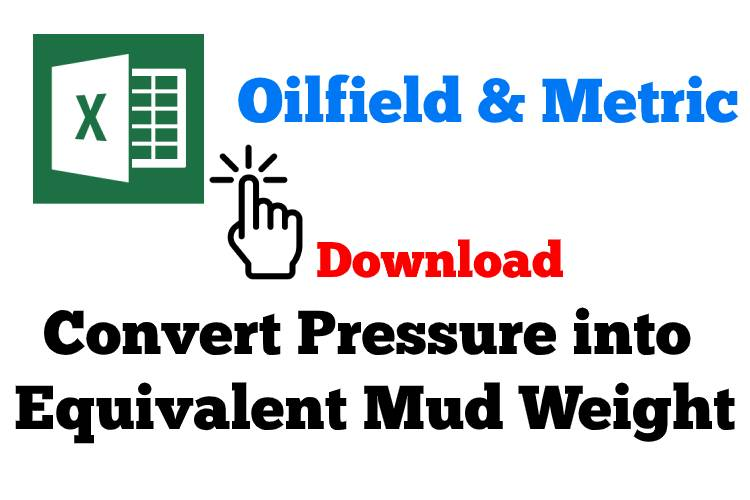# Convert Pressure into Equivalent Mud Weight

Learn how to convert pressure into equivalent mud weight into equivalent mud weight as per following formulas.# Oilfield Unit

## Convert pressure in psi  unit into equivalent mud weight in ppg by using feet as the unit of measurement

Equivalent Mud Weight in ppg = pressure in psi ÷ 0.052 ÷ True Vertical Depth (TVD) in ft

Example:

Pressure = 5,000 psi
True vertical depth = 8,000 ft
Equivalent mud weight, ppg = 5,000 psi ÷ 0.052 ÷ 8,000 ft
Equivalent mud weight, ppg = 12.0 ppg

# Metric Unit

## Convert pressure in KPa unit into equivalent mud weight in Kg/m3 by using meters as the unit of measurement

Equivalent Mud Weight in Kg/m3 = Pressure in KPa × 1000  ÷ True Vertical Depth (TVD) in meters ÷  9.81

Example:

Pressure = 35,000 KPa
True Vertical Depth = 2,500 meters
Equivalent mud weight, kg/m3= 35,000 × 1,000 ÷ 2,500 ÷ 9.81
Equivalent mud weight, kg/m3= 1,427  kg/m3

Please find the Excel sheet for converting pressure into equivalent mud weight.Ref book:

Lapeyrouse, N.J., 2002. Formulas and calculations for drilling, production and workover, Boston: Gulf Professional publishing.

Bourgoyne, A.J.T., Chenevert , M.E. & Millheim, K.K., 1986. SPE Textbook Series, Volume 2: Applied Drilling Engineering, Society of Petroleum Engineers.

Mitchell, R.F., Miska, S. & Aadny, B.S., 2011. Fundamentals of drilling engineering, Richardson, TX: Society of Petroleum Engineers.

Share the joy
Tagged . Bookmark the permalink.